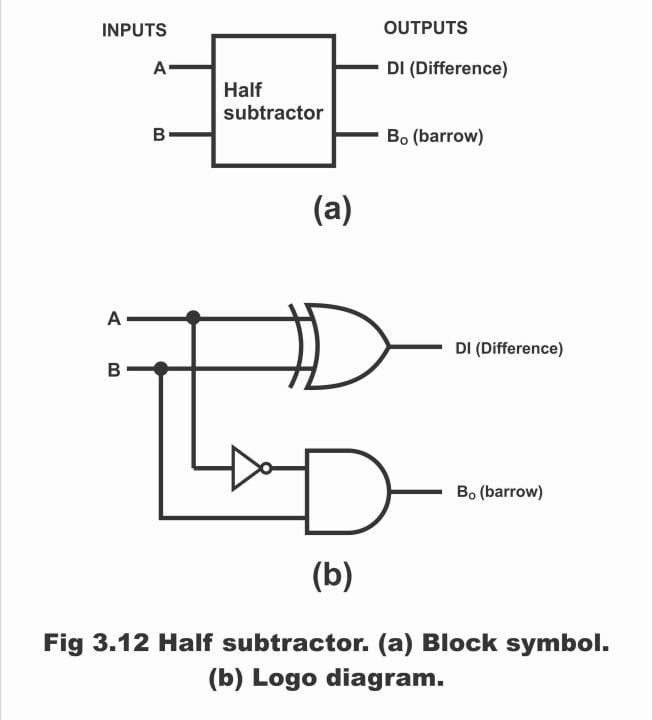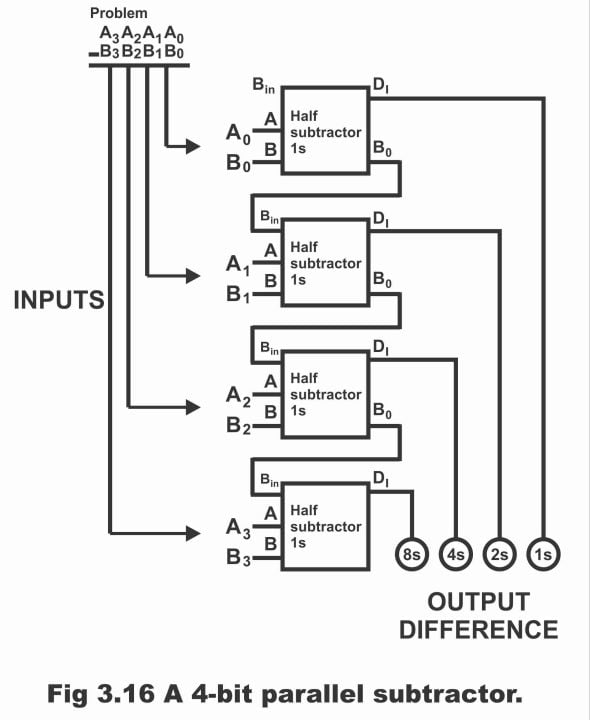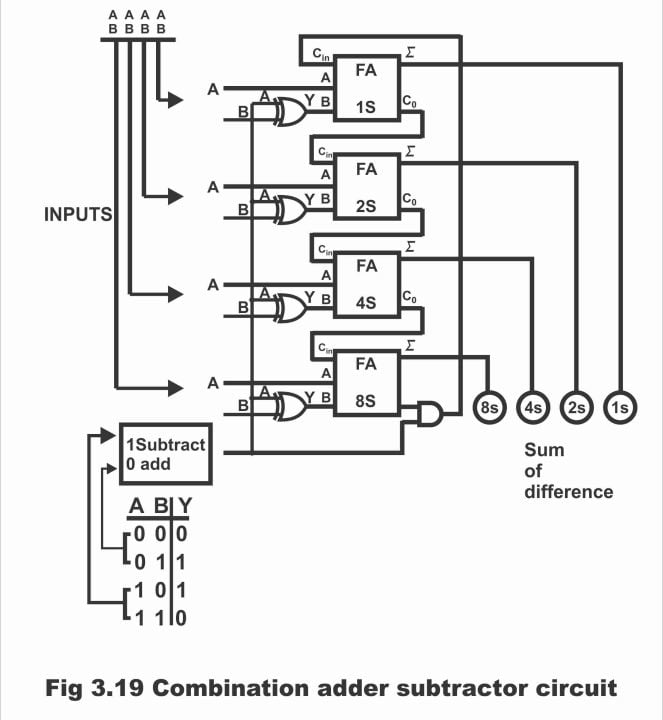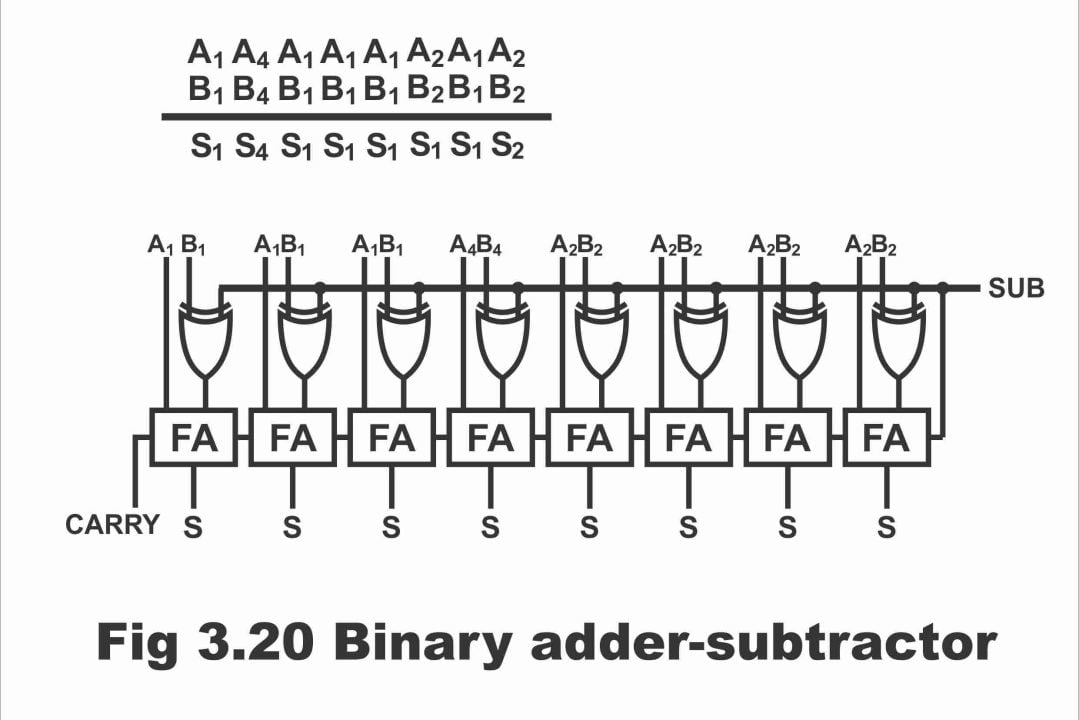Digital Electronics

# Half subtractor and Full subtractor with Equations in Digital Electronics

## Binary Subtraction

Half subtractor and Full subtractor with Equations in Digital Electronics– Similar to the addition of binary digits, following four rules or principles are used as well for the subtraction of binary digits.

0 – 0 = 0

1 – 1 = 0

1 – 0 = 1

10 – 1 = 1 0 -1 with a borrow of 1

During the subtraction of digits, we have sometimes to borrow a digit from the next left column. This borrowed digit is shortly known as Bo (borrow out). In case of binary, we need a borrowed digit at that time when we attempt to subtract 1 from 0. In such a situation, when 1 is borrowed from the next column towards the left, it becomes 10 in the column where it is going to be subtracted. And for this end, last rule of the afore – mentioned four principles is used.

The phenomenon of a binary subtraction can easily be grasped with the help of following example.

Example: – perform the following binary subtractions

(a). 11 – 01 (b). 11 – 10 (c). 101 – 011

Solution: –

(a). 11 – 01 = 10     3 – 1 = 2

(b). 11 – 10 = 01     3 – 2 = 1

(c). 101 – 011 = 010           5 – 3 = 2

## Subtractors

A logic circuit, which is used to subtract two binary numbers, is called subtractor. Similar to an adder, a subtractor also has following two types.

1. Half–subtractor
2.  Full–subtractor

### Half – subtractor

It is a kind of logic circuit, which has the capacity to subtract two binary digits or bits at the same time and which has two outputs. One of the outputs represents the inputs difference (Di) while other one denotes Borrowed or Bo.

In figure 3.12 (a), block diagram or block symbol of a half – subtractor and in figure (b) its logic diagram has been illustrated. It is clear from the block symbol that a subtractor consists of two inputs (A and B) and two outputs (Di and Bo). Its inputs are on the left whereas its outputs are located on the right side.

Figure 3.12 – half subtractor (a). block symbol (b). logic diagramIn figure 3.13 (a), a binary subtraction table and in figure (b) truth table of a half – subtractor has been shown. In this, B has been subtracted from A on the input side. Similarly, on the output side, we get difference of inputs A and B or Di. If the value of B is higher as compared to A, as can be observed via line 2 of the truth table, in such a situation we will need to borrow one. This has been explicated on the output side by column Bo (Borrow out)

Figure 3.13 – (a). binary subtraction tables (b). truth table for the half – subtractor

With the help of a truth table (which reflects all inputs and outputs) shown in the figure3.13 (b), we can determine the Boolean expressions of a half – subtractor. Remember that operation of a half – subtractor depends on the rules of binary subtraction as has been shown by the truth table. As the difference column represents a difference 1 in case both of the inputs are inverse to each other (analogous to Ʃ column of the half adder). Thus, a XOR gate is used in order to ascertain difference between two bits and the expression of difference or Di just like a half – adder is denoted as follows:

AꚛB = Di

Whereas borrowed output (Bo) can be received via ANDing A with B.  Hence, Boolean expression of the borrowed (Bo) column will be as follows:

1. B = Bo

If above two equations are combined together in the shape of a logic diagram, a logic circuit of a half – subtractor constructs as has been shown vide figure 3.12 (b).

### Full subtractor

A logic circuit comprising three inputs and two outputs, which can simultaneously subtract two bits, is known as full – subtractor. In figure 3.14 (a), block diagram of a full – subtractor has been represented. On the left side of this diagram, three inputs A, B and Bin (Borrowed input) are present, whereas on the right side, two outputs Di and Bo exist. Bin is in fact a “previous borrow” on the input side.

Figure – full subtractor (a). block symbol (b). constructed with half subtractors and an OR gate (c). logic diagramJust like full adders, a full subtractor can also be constructed by means of using two half – subtractors and an OR gate, as has been represented via figure (b). Logic diagram of a full subtractor has been depicted in figure (c).

As a sample, binary subtraction has been illustrated in the figure 3.15 (a). When subtraction of a number of columns of binary digits is desired to be undertaken, in this situation borrowing factor should particularly be kept into mind. In figure (b), truth table of a full – subtractor has been demonstrated, which represents all the possibilities of binary subtraction (A-B-Bin). The method for understanding the truth table of a full – subtractor is as under:

Figure 3.15 – (a). sample binary subtraction problem (b). truth table for a full subtractor

Look at line 2 of the table, wherein A = 0, B = 0 and Bin = 1. For going ahead with subtraction process, we have to borrow 1 from the next stage, by means of which Bo = 1 and 2 is added with A. Thus, Di = 1 (because 2-0-1=1). When A = 0, B = 1 and Bin = 1 (line number 4), we again feel the need to borrow, due to which Bo = 1 and A = 2. Hence, 2 -1 -1 = 0, thus Di = 0. Similarly, when A =1, B = 0 and Bin = 1, (see line number 6) then, A-B-Bin = 0. As a result of which, Bo = 0 and Di = 0. When A = 1, B = 1 and Bin = 1 (last line of the table), we have to borrow 1 once again, hence Bo = 1 and A = 3, thus Di = 1. (i.e. 3-1-1 =1)

Boolean expressions of full subtractor are as under:

Di= A B Bin + A B Bin + A B Bin + AB Bin

Bo= AB + A Bin + B Bin

Remember that in full adder and full subtractor, the logic function for sum S and difference Di is identical. Moreover, it must also be inculcated that through cascading (giving ones’ output to the others’ input is known as cascading) 4 full subtractors, we can directly subtract 4-bit numbers i.e. we can subtract B0 B1 B2 B3 from A0 A1 A2 A3, which has been shown in figure 3.16.

Figure 3.16 – A 4- bit parallel subtractorA logic circuit, which apart from addition, can also perform the function of subtraction, is called adder – subtractor. We have studied addition subtraction detail of binary numbers prior to this, wherein numerous logic circuits have been used for addition and subtraction of various binary numbers. In order to simplify circuits for arithmetic operations in calculating machines (e.g. calculator or computer etc.), attempts are underway to use some type of universal devices, through application of which process of calculations or arithmetic operations becomes simpler and accurate. For this purpose, such circuits are being designed via application of specific techniques, which can be used both as an adder and subtractor (i.e. in this way, the task of addition and subtraction through one circuit becomes possible).

There is a special technique being used in mathematics through which an adder can be used for the subtraction of binary numbers. This technique has been shown in figure 3.17. According to this figure, 6 has been shown as being subtracted from decimal 10 or in case of binary, 0110 being subtracted from 1010. In the figure, first of all decimal subtraction, then binary subtraction and lastly, special arithmetic technique has been shown, by means of which, an adder converts to a subtractor.

Figure 3.17 – an example of 1s complement and end – around carry subtraction

Although the method of 1’s complement and end- around carry apparently seems lengthy and somewhat complicated, however through application of logic circuits, the subtraction function can be undertaken quite easily by means of application of this technique. In this method, an adder is used, which performs the operation of subtraction. The only issue lies in the fact that we have to ascertain as to how to carry out the process of subtraction by means of taking 1’s complement and the end around carry.

### Operation

Figure 3.18 – using full adders and inverters to construct a 4 – bit binary subtractorThe following subtraction can be explained from the 4 – bit binary subtraction circuit

A0 A1 A2 A3

-B0 B1 B2 B3

Ʃ0 Ʃ1 Ʃ2 Ʃ3

Suppose we have to subtract decimal 4 from decimal 10 or in case of binary we have to subtract 0100 from 1010 i.e.

Decimal subtraction                                        Binary subtraction

10 – 4 = 6                                                             1010 – 0100 = 0110

In such a situation, A0 A1 A2 A3 and B0 B1 B2 B3 inputs are as follows:

A0 A1 A2 A3          →            1010

B0 B1 B2 B3            →            0100

We replace inverter B present in the circuit with the under – noted 1s complement

B0 B1 B2 B3            →            1011

Thus, full adder performs the following functions

1010

+1011

0101

+1       end- around carry

0110

If light emitting diodes are connected with the output (which reflects the difference) of this circuit made with the help of full adder and inverter, in such a situation LED of 1’s will not irradiate (which reflects low output). However, 2s and 4s LEDs get illuminated (which indicate a high output) Resultantly, subtraction answer becomes clear once we look at the LEDs output.

Thus, subtraction answer on the output will appear as 0110 which is equivalent to decimal 6.

We know that a full adder can be used both for addition as well as subtraction purposes (i.e. addition and subtraction operations can also be performed within a circuit along with a common binary adder). This objective can be achieved via adding a XOR gate with every full adder. Now we design such a system, which can process both addition as well as subtraction functions.

First of all, we begin from the subtractor system shown in the figure 3.18. In order to convert this system into a 4 – bit adder, we will need to cut end around carry line and doing away with the 4 inverters, as can be seen in figure 3.19. According to this figure, 4 XOR gates have been mounted in place of 4 inverters. When input A of XOR gates is binary 0, problem bits pass through the XOR gate without any variation (i.e. when input A is zero, in such a situation the two numbers which are required to be added, its bits pass via XOR without any change), as has been revealed via a table shown below the figure. Thus, by means of setting control at 0, adder – subtractor unit, adds binary number A0 A1 A2 A3 with B0 B1 B2 B3 and result appears on output indicators (up to a sum of 1111). Remember that by setting control on zero (i.e. setting control on addition state) AND gate also turns OFF or AND gate ceases to operate. Thus, connection of end around carry suspends with the first full adder or end around carry signals stop transmitting from AND gates’ output.

When the unit shown in the figure is going to be used as a 4 – bit subtractor, its control must essentially be at position 1. In this situation, XOR gate acts as an inverter for B inputs leading to the full adder, as can be seen via the truth table towards the left on bottom of the figure.  AND gate also activates due to having 1 on control (that’s AND gate starts working) and as such information is transmitted back on full adder 1s in the shape of end around carry from full adder 8s. This subtractor subtracts binary input numbers B0 B1 B2 B3 from A0 A1 A2 A3 and difference appears on output indicators in the shape of binary. Remember that in this circuit, 1s complement and end around carry method is used for the purpose of subtraction and XOR gate acts like a complement.

Figure 3.19 – combination adder – subtractor circuit## 8 – Bit Adder – subtractor

In figure 3.20, an 8 – bit binary adder – subtractor circuit has been illustrated, which adds or subtracts binary numbers. This circuit is spread across from left to right, i.e. the least significant column of the circuit is towards right whereas the most significant column is towards the left. The carry – out of every full adder (FA) is the carry – in of every next full – adder. Remember that during 8 – bit arithmetic operation, final carry is ignored (because in case of 8 – bit mathematical operation, values remain between 0 – 255, therefore every number supposed to be added or subtracted, must remain between 0 – 255. Further, the answer should also be within a range 0 – 255. If values exceed 255, the 16 – bit arithmetic operation should be used. While using the 8 – bit arithmetic operation, if the answer as a result of addition is greater than 255, an overflow occurs and a carry moves into the 9th column. There is just one circuit in most of the microprocessors, which is known as carry flag. This circuit, provides information sensing carry in the 9th column, that 8 – bit answer is invalid. That’s the reason, final carry is generally being ignored during an 8 – bit arithmetic operation)

Figure 3.20 – binary adder – subtractorThe function of addition is represented as under:

A0 A1 A2 A3 A4 A5 A6 A7

+ B0 B1 B2 B3 B4 B5 B6 B7

S0 S1 S2 S3 S4 S5 S6 S7

For example, suppose that +125 and -67 are required to be added, then the values of A0 – A7 and B0 to B7 are equal to the following:

A7           –              A0 =        0111       1101

B7            –              B0 =        1011       1101

As SUB = 0 during the addition operation, therefore the value carry – in of least significant column is zero i.e.

0       ←            SUB

0111     1101

+1011    1101

?

Here, full – adder performs the following arithmetic operation

0 +1 +1 = 0           with a carry of 1

Carry out of first full adder is carry in of the second full adder i.e.

1          ←            carry

0111    1101

+1011   1101

0

By adding in the 2nd column,

1 + 0+ 0 = 1          with a carry of 0

0           ←            carry

0111     1101

+1011    1101

10

Similarly, remaining full adders add their 3 – input bits, until we arrive at last full adder. Hence

1             ←            carry

0111     1101

+1011    1101

0011     1010

In case the most significant bit (MSB) lying on the left end, receives carry – in, then full adder presents the following answer to the addition

1 + 0+ 1 = 0 with a carry of 1

After this final carry, the function of addition gets completed i.e.

0111     1101

+1011    1101

10011    1010

As this final carry is ignored in an 8 – bit arithmetic operation, thus addition answer is as under:

S7 … S0 = 0011     1010

This answer is equivalent to decimal +58, which is a sum of numbers +125 and -67

ii). Subtraction

The process of subtraction is represented through the following method.

A7 A6 A5 A4 A3 A2 A1 A0

-B7 B6 B5 B4 B3 B2 B1 B0

S7 S6 S5 S4 S3 S2 S1 S0

Remember that the SIB signal is kept on high state during subtraction, as a result of which 1’s of control inverter B0 to B7 produces complement. Moreover, as SUB signal is received on the full adder as Carry – in, therefore circuit process data in the following method

1        ←            SUB

A7 A6 A5 A4 A3 A2 A1 A0

+B7 B6 B5 B4 B3 B2 B1 B0

S7 S6 S5 S4 S3 S2 S1 S0

When A7 … A0 = 0, circuit produces 2’s complement of B7 … B0, which is added into the 1’s complement of B7 … B0. (when 1 is added to 1’s complement, the binary number received as a result of this operation is called 2’s complement i.e.

(2’s complement = 1’s complement +1). When A7 … A0 are not equal to 0, then effects A7 … A0 and B7 … B0 equal to the sum of 2’s complement.

For example, suppose that we have numbers +82 and +17. In such a situation,

A7 … A0 = 0101   0010

B7 … B0 = 0001    0001

Control inverter B’ s 1’s produces complement which is 1110       1110. As during subtraction, SUB = 1, hence circuit performs the following function of addition

1←SUB

0101     0010

+1110    1110

10100    0001

As final carry is ignored in an 8 – bit arithmetic operation, therefore the answer becomes

S7 … S0 = 0100     0001

This answer is equal to decimal +65, which is the arithmetic difference found between numbers +82 and +17.

For electronics and programming-related projects visit my YouTube channel.